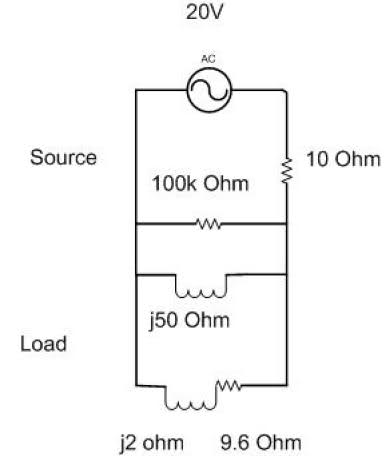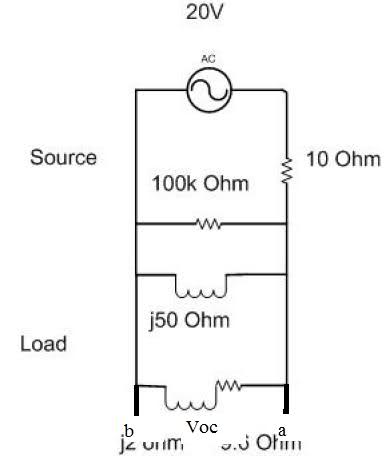# Thevenin equivalent q. (AC analysis)

• eeuler
In summary, the conversation is about finding the Thevenin's equivalent circuit for a given source. The person is unsure how to approach the problem and asks for clarification on whether to consider the load as part of the circuit or not. The expert explains that the load must be taken off the circuit to calculate the open circuit voltage, and there is current passing through the 100k resistor and j50 inductor. The person then realizes their mistake and thanks the expert for their help.

## Homework Statement^We're asked to find the "Thevenin's equivalent circuit for the source"

## The Attempt at a Solution

I'm a bit unsure how to start this problem. What i initially thought was that i'd combine all my impedances in parallel to the point where it's them combined plus the 10ohm resistor in series, then use the voltage divider..however the part of the circuit labeled as 'load', well am i suposed to find the voltage across the inductor and resistor in series (9.6+j2ohms), as in, is that where my Voc is? And thus the 10ohm resistor is useless in calculating the Thevenin voltage (since the current entering it would be zero)?

Is that how i should approach it? Consider Voc to be where the resistor and inductor are? I don't think i explained myself properly, but this is what i mean:Just that in other (similar) problems I've done, they either have just the open circuit nodes a and b (with the rest of the circuit being to the left) or they have an arbitrary load impedance (ZL) connected to a and b nodes (with no value). So am i to find the open circuit voltage between terminals a and b (including the resistor and inductor in series)?

The 9.6+j2 Ohm load must be taken off the circuit in order to calculate the open circuit voltage. The voltage is divided between the 10 Ohm resistor and the parallel of 100k and j50 Ohm.
Why do you say that there is no current through the 10 Ohm resistor?

^^Thanks for the reply, but i figured it out :)

I thought that there'd be no current through the 10ohm resistor, because when you're calculating the Voc, the component on the horizontal branch that leads to one of the open terminals, well isn't the current through that branch zero..?

eeuler said:
^^Thanks for the reply, but i figured it out :)

I thought that there'd be no current through the 10ohm resistor, because when you're calculating the Voc, the component on the horizontal branch that leads to one of the open terminals, well isn't the current through that branch zero..?

No, because there is current through the 100k resistor and the j50 inductor.